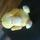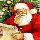## General Question# What brand of calculator goes to the highest number?

Asked by RedDeerGuy1 (15870) 2 weeks ago

Before saying error. My ti-85 goes to 109999 or 10999. What goes highest? Smart phones included, but prefer a straight up calculator.

Observing members: 0Composing members: 0I had calculators that converted the number to exponential.
Numbers with explicit powers of ten (such as 4.2×10–5) are displayed with an ‘E’ preceding the exponent. Thus 4.2×10–5 is entered and displayed as 4.2E-5. A number whose magnitude is too large or too small for the display format will automatically be displayed in exponential form.

Tropical_Willie (25227)“Great Answer” (1) Flag as…I ran the following python program on my PC: math.log10(math.factorial(10000)**3)
The number returned was a little over 100,000, meaning that the program was able to handle numbers over 10**100000. The program returned the answer immediately. I will have to see at what point the computer complains about the size of the number.

I just tried math.log10(10**1000000). It took a few seconds to run, but the return value of one million is correct.

LostInParadise (26485)“Great Answer” (1) Flag as…The fun thing about computing large numbers using Python on the PC is that you can see all the digits. For example 300! (pronounced 300 factorial and is what you get when you multiply together all the numbers from 1 to 300) in Python is math.factorial(300), using the math library. If you do this, you will see a number taking up a few lines ending with a whole bunch of zeros at the end,

Here is a fun math exercise. Just how many zeros are there at the end of 300! ? You should be able to solve this in your head,

LostInParadise (26485)“Great Answer” (1) Flag as…The answer is 74 zeros. The number of zeros equals the number of tens in the factorization of 300!. Consider the prime factorization. You get a 10 whenever you combine a 5 and a 2. There are going to be a lot more 2’s than 5’s, so the question is how many 5’s there are.

Every fifth number is divisible by 5, so there are 300/5 = 60 numbers that contribute at least one 5. There will be 300/25 = 60/5 = 12 numbers that contribute a second 5. Finally, there are two numbers, 125 and 250 that contribute a third 5. 60 + 12 + 2 = 74.

LostInParadise (26485)“Great Answer” (0) Flag as…or